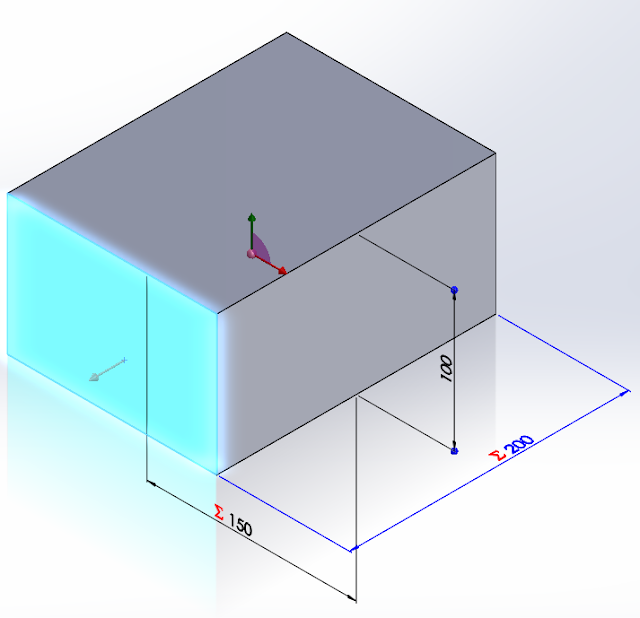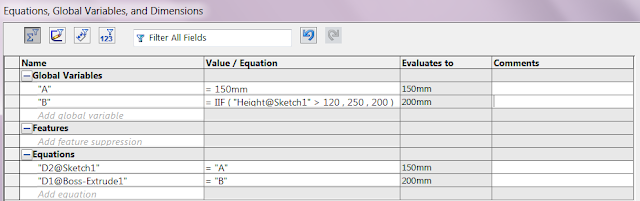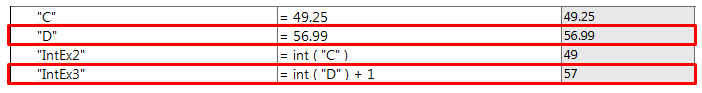Using SOLIDWORKS Equations

SOLIDWORKS Equation features is a powerful and convenient feature to allow users to define dimensions using global variables and mathematical functions, and furthermore create mathematical relationships between two or more dimensions in your part and assembly designs.

However, most of the time is that users are only using the most basic capabilities of SOLIDWORKS Equation without realizing the advanced operators that are available. As such, this article introduces some of the operators or Visual Basic key words.

A commonly used operator would be the “IF” Operation, where users are able to include a “If, Then, Else” mathematical condition to their designs.

The example below shows a simple example of the “IF” Operation usage.

This cube has been constructed with a height of 100mm, width of 150mm and a length of 200mm by including the global variable as below:

A = 150

B = 200For this example, lets control the design so that when the height of the cube exceeds a certain number (>120mm), the length of the cube will be increased to 250mm instead.

To do so, we have to go to the Equations, Global Variables, and Dimensions window. Under the B global variable, instead of inputting 200mm, we are going to input the “IF” Operation which will have the format:

DimensionValue = IF(ConditionValue>X, ThenValue, ElseValue)

Based on our condition for the cube, our input for the formula will be as shown. When constructing the equation, the ConditionValue will be an input by selecting the dimension from the graphics area of SOLIDWORKS.This equation dictates that if the height of the cube exceeds 120mm, the length of the cube will increase to 250mm, or else if the height is below 120mm, the length will remain as 200mm.

Let’s try to change the height from 100mm to 130mm and see if our equation works.We see how it will automatically update the length to 250mm. Now let’s try a height of 110mm and see what happens.Another useful tool that users can benefit from is the integer, int() function and the round, round() function. Let’s take a look at the integer, int() function first. Here we are going to add in this function into our global variable. As seen in the figure below, you will notice that the integer function rounds DOWN any value with decimal places to the nearest whole number.So what about the round function? What the round function does in SOLIDWORKS is that it will round up any values with decimal places to the nearest whole value, UPWARDS or DOWNWARDS. Anything below a decimal place of 0.5 will be rounded down and anything equal 0.5 or above will be rounded up. For example:Let’s add a few round, round() functions into our example. The E and F values will be rounded up or down depending on the decimal places.But what happens if we want our decimal values to always round UPWARDS only? Although SOLIDWORKS equations does not have a direct function that allow users to do that, there is a workaround to go about solving this tricky situation. All we need to do is to add a “+1” after our integer, int() function. Let’s do that by revisiting the existing example that we have here:As such we are able to have the “IntEx3” Global Variable to always round up to the closest value, in this case for 56.99, rounding it downwards to 56 using the integer function and then adding 1 to make it 57.  But of course the few functions introduced here are not the only ones available in SOLIDWORKS, so do continue exploring the other capabilities of SOLIDWORKS Equation!

Prepared by JJ Chan,

Application Engineer, CADVision Systems Sdn. Bhd.

p/s: Suggest us a topic that you would like to discover by sending us an email to eventpg@cad-vision.com